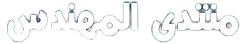# How to find the FFt and angle of this function

salam to all
how to find the FFT and angle of this function using matlab

w=2pi50;
c=1
step=0.001;
period=0.02;
t=0:step:c*period;

z= (5)…
+(80sin(wt))…
+(1cos(2wt))…
+(8
sin(3wt+2.88))…
+(1cos(4wt))…
+(4
sin(5wt+2.76))…
+(1cos(6wt))…
+(1
sin(7wt+2.62));